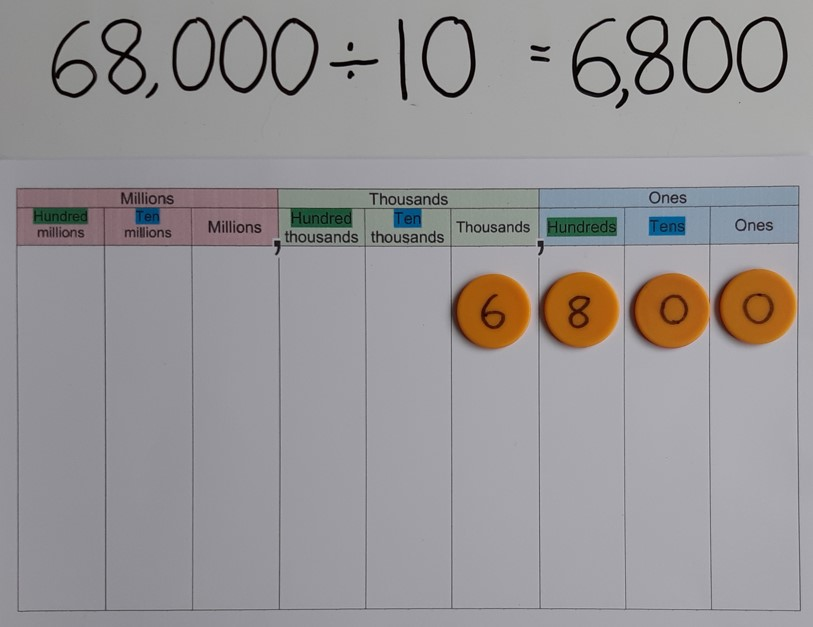## Dividing by 10

When a number is divided by 10, each part of that number becomes 10 times smaller. So a hundred becomes a ten, and a ten becomes a one. This can easily be seen using Dienes, reversing the process used when multiplying by 10, with a hundred flat becoming a ten stick and a ten stick becoming a one cube. This means that any placeholder in the ones position is no longer needed. If there was a digit in the ones place, this will move into the tenths place to give a decimal answer.Using a place value grid, we can see that the digits move one place to the right as they are divided by 10, representing how the value of each has become 10 times smaller. The digits move the opposite way to when we multiply by 10, because division is the inverse, or opposite, of multiplication.Download an activity pack of games to reinforce multiplying or dividing by 10, 100 or 1000
Click here to generate worksheet to practise multiplying and dividing by 10, 100 & 1000.